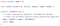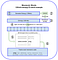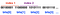# Analyzing Trezor Firmware: Mnemonic Seed Generation for Bitcoin and Ethereum

Trezor’s firmware is open-source. Its crypto library is used widely by companies like TrustWallet for generating wallets in Bitcoin and Ethereum. Let’s examine how the Trezor crypto library generates mnemonic seed phrases for Bitcoin and Ethereum.

If you’re not already aware, mnemonic seed phrases pull from a well-defined wordlist containing 2048 words. Secure mnemonic phrase generation is a function of how you index into that wordlist to select words from it. Since there are 2048 words, you need 11 bits to reach every possible word.

The first function the Trezor firmware calls to generate a mnemonic seed phrase is mnemonic_generate(), which accepts a strength (the amount of entropy to encode, where 256 bits produces a phrase of 24 words). This function ultimately returns the character array which is your mnemonic seed phrase.

uint8_t data gets passed to the random_buffer() function, whose job is to populate it with randomly generated bytes.

The random_buffer() function looks as follows, where uint8_t *buf is our data array, and size_t len its length (32).

Since each index in the array stores 8 bits, every 4 indices store 32 bits. The for loop shows that that every 4 indices into the array, we assign variable r the return value of the random32() function, whose job it is to return a random 32-bit value.

This random32() function is the last function called in the Trezor library for generating randomness. Whatever random32() does — whether it returns the same 32 bits every time, or pulls from a cryptographically secure random number generator — is what will be used to select words for the BIP39 wordlist.

By default, the rand.c file containing the random32() function states:

Thus, the Trezor library mandates you, the user of the library, to supply your own source of randomness by overwriting the function definition of random32().

To this effect, the default implementation of random32() in the Trezor firmware repository is not suitable for production use. It produces predictable numbers, yielding the same seed phrase every time.The default implementation of random32(), left to the user to change.

Assuming you replace this function with a cryptographically secure random number generator, we can re-examine the random_buffer() function:

The line at the bottom copies the random 32-bit value assigned to `r` into the buff array one byte at a time.

Let’s walk through one iteration:

When `i = 0` , `r` (the random 32-bit value) is right shifted by `((i % 4) * 8)` bits, which is`((0) * 8)` or 0 bits. The result of that is `AND`'d with `0xFF` , which always results in the last byte of whatever you are`AND`’ing being kept.

Thus, when `i = 0`, the last byte of that random 32-bit value is placed in index 0 of the buf array. When `i = 1`, that 32-bit random value is right shifted by 8 bits, then `AND`’d with `0xFF`. This means `buff` will contain the second-to-last-byte of that random value. This continues until the full 32-bit value is stored in indices 0–3, and a new random 32-bit value is picked when `i = 4`.

After random_buffer() returns the array of random 32-bit values, we proceed to mnemonic_from_data(), which accepts that array and its length (32).

This function first creates a byte array called bits of length 32 + 1 (33). This array, along with the data array and its length, get passed to the sha256_Raw() function, which generates a SHA256 hash of the random bytes in the data array and places it inside of the newly created bits array.

After that function returns, you see the line:

You’ll notice the bits array is of size 32 + 1. That 33rd byte (index 32 in the array) becomes part of the data used to select the 24th word of the mnemonic phrase.

When generating a 24 word mnemonic seed phrase, the 24th word is not selected like the previous 23. Instead, part of it comes from a checksum of the other 23 words. Specifically, the first byte of the checksum of the 32 random bytes we generated gets appended to the end of that array. To that effect, `bits[len]` gets assigned the value of `bits`, which is the first byte of the checksum.Diagram showing how the checksum is appended, from Andreas Antonopolous’s “Mastering Ethereum”

The next line copies the 32 bytes of random data from the data array into the first 32 indices (indices 0 through 31) of the bits array.

Now, the bits array contains the random 32 bytes we generated earlier in indices 0–31, and the first byte of their checksum in index 32. This is 33 bytes total, which is also 264 bits.

As I mentioned earlier, we need 11 bits to be able to select any word from a wordlist containing 2048 words. Dividing the 264 bits of data we have from the 11 bits-per-word requirement gets you 24 words . Thus, we have all the data we need to create our randomly-selected 24 word mnemonic phrase.

The next section in this function is how the Trezor firmware converts our bits array into indices in the wordlist:

The Trezor team named the byte array bits because it is treated as a grouping of 11 bits at a time. Each 11 bits represents one index into the BIP39 wordlist.

The outer loop goes from 1 to mlen, which was declared earlier as the length of our mnemonic phrase, 24. The inner loop iterates 11 bits at a time, converting them into an integer value by growing the variable `idx`. That variable is then used to select a word from the wordlist.

When `i = 0` (that is, we’re selecting the first word of our mnemonic), `idx` is first set to 0. In the inner loop, `j = 0`, meaning we are evaluating the first bit of the first 11 bits in the bits array.

The first thing we see is `idx <<= 1`. This is a left shift assignment operator, which means to left-shift `idx` by one bit, and then store the result back in `idx`. A simpler explanation: you are multiplying `idx` by 2. I’ll explain why this is being done shortly.

The next line is more involved. Before reading any further, understand that the reason this next line exists is because we are trying to examine an array where each index is only 8 bits long 11 bits at a time.

The bits array might look as follows:

But, since we want to treat this array as groupings of 11 bit indices, a full index spills across multiple elements in the bits array:Considering 11 bits at a time from the byte array called bits

Keeping that in mind, the line is:

The first part of this equation is `bits[(i * 11 + j) / 8 ]`. This returns the byte in the bits[] array that contains the specific bit we are examining. Here’s how that works:

Since each index is represented by 11 bits, `i * 11 + j` represents the “absolute index” of the bit being examined. There are 264 total bits in this array. If `i = 0` and `j = 5`, this portion of the equation returns `bits[(0 * 11 + 5 / 8)] = bits[5 / 8] = bits` , the byte where the 6th bit lives.

We `AND` that specific byte with the result of the right side of the `&` operator, which creates a different byte from scratch.

This new byte being created on the right side of the `&` will be all `0`'s except for the specific bit we are examining, denoted by `j`.

The right side of the `&` looks as follows:

This takes the absolute index of the bit in `i * 11 + j`, finds out how far into a specific byte it would be by doing `% 8`, then subtracts this value from 7 and right-shifts `1` by that many bits. The intention is to find how many `0` bits need to be added to the right of this `1` bit to create a new byte that has that specific `1` bit lining up exactly where the bit we are examining in the byte returned from the left side of `&`.

We saw how plugging in `i = 0` and `j = 5` returns `bits` on the left side of the `&`. Let’s assume `bits` is `0b00101000`

Plugging in those `i` and `j` values into the right side of the `&` gets you:

We created a new byte from scratch, with a `1` bit in index 5 (the 6th bit in the byte).

Now, we can finally `&` the left and right sides:

The left side was `0b00101000`. The right side is `0b00000100`.

If this `AND` operation produces a byte of all `0`s, we know that bit was not set in the bits array (the byte being examined in the left side of the `&`). If the result is not all `0`s, we know that a `1` was present at that location in the bits array.

In our case, that result was not all `0`s, meaning the bit being examined from the bits array was set to `1`. The result of this `&` is a value greater than 0, meaning the final check from the far right of the equation `> 0` evaluates to 1 (or true).

Since the `> 0` check evaluated to 1, `idx` gets incremented by 1. The inner `j` loop proceeds for each remaining bit in the 11 bits we are considering.

The line `idx <<= 1` from earlier should make more sense now. Every time a bit is present in the bits array, 1 is added to `idx`. That `1` needs to go into its appropriate location within `idx`.Considering 11 bits at a time from the byte array called bits

If the 0th bit of the 0th word is set to `1`, then that `1` really represents 2¹⁰. If the 3rd bit is set, like in the image above, it really represents 2⁸. This `idx <<= 1` converts the binary representation of these 11 bits into an integer by using the inner `j` loop to multiply `idx` by 2 as many times as needed.

After the `j` loop terminates, the final step is:

This takes the integer produced from the 11 bits and uses it to index into `wordlist`. That word gets copied to `p`, a pointer to the start of the character array declared earlier to store the mnemonic.

`p` gets incremented by however long that word was in the line `p += strlen(wordlist[idx])`. If this was not the 24th word, a space ( `' '`) is added to `p` to visually separate this word from the next word in the mnemonic when it gets returned to the user. If it was the 24th word, a `0` is added, denoting the end of the string.

After `i` and `j` build 24 indices by iterating over this array 11 bits at a time, the mnemonic phrase is generated returned to the user.

# Conclusion

The path I outlined in this post works as expected. With that said:

• It is ill-advised to provide a default implementation of a cryptography library that is completely insecure. The git history shows that random32() at one time pulled from `/dev/urandom`, and another time used a predictable timestamp as the seed. Throwing an exception is the only correct option here, regardless of allowing users to “test” the library out of the box.
• The code I showed to generate 11-bit indices is wildly inefficient. It does tens of operations to iterate bit-by-bit what could easily be done word-by-word in memory, masking 11 bits at a time. This is strangely juxtaposed with explicitly using a left-shift assignment operator, when any modern compiler would optimize a more readable multiplication by 2.

The git history was interesting to look through. I encourage you to read through it yourself.